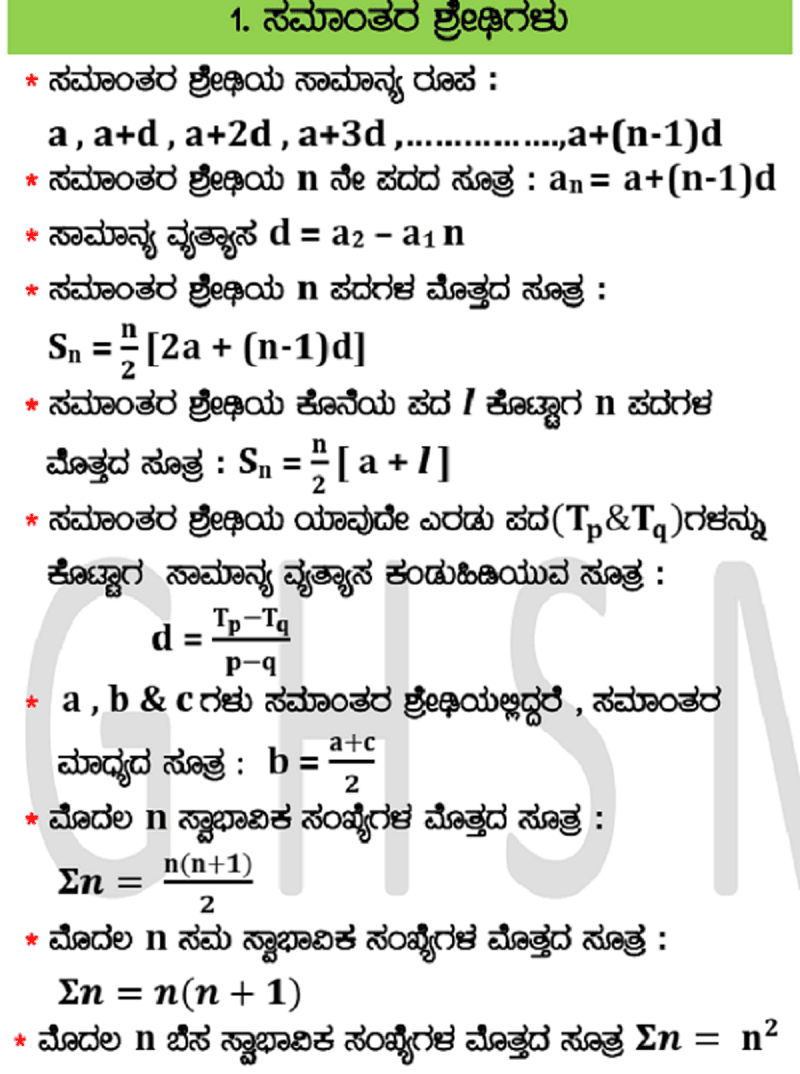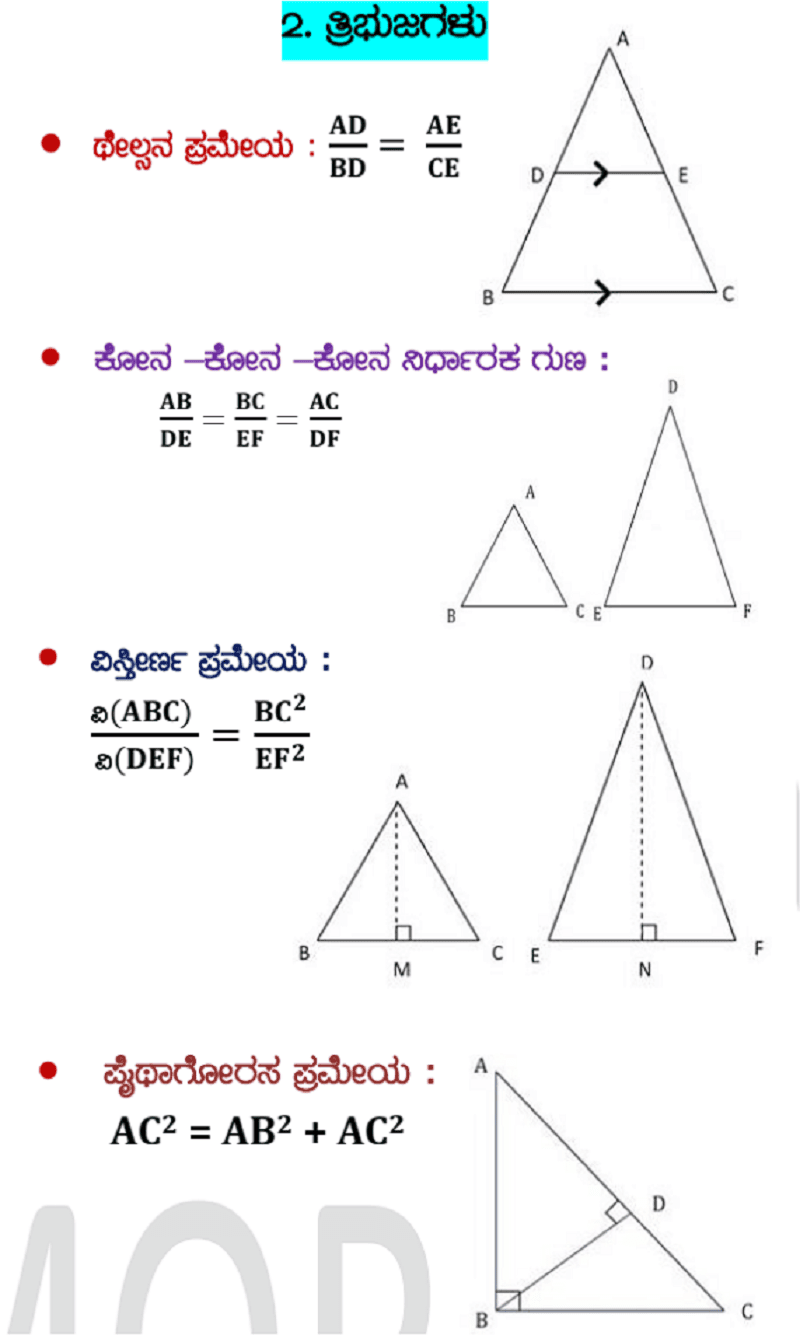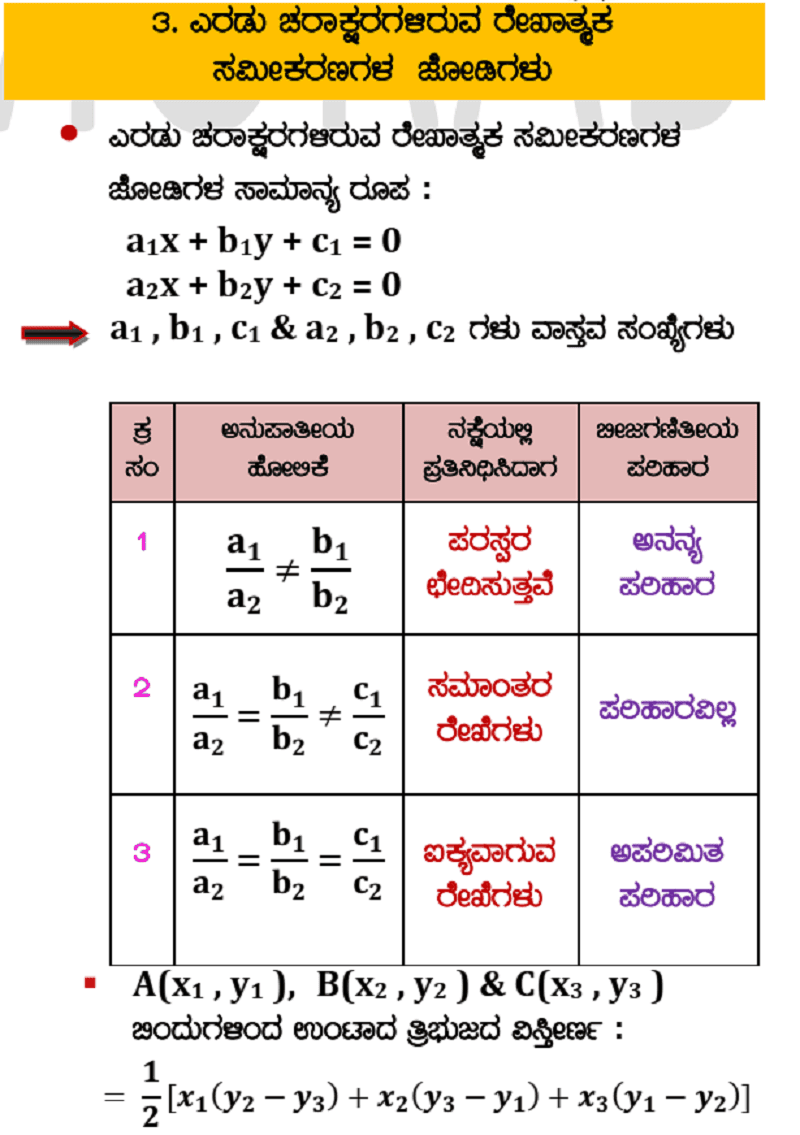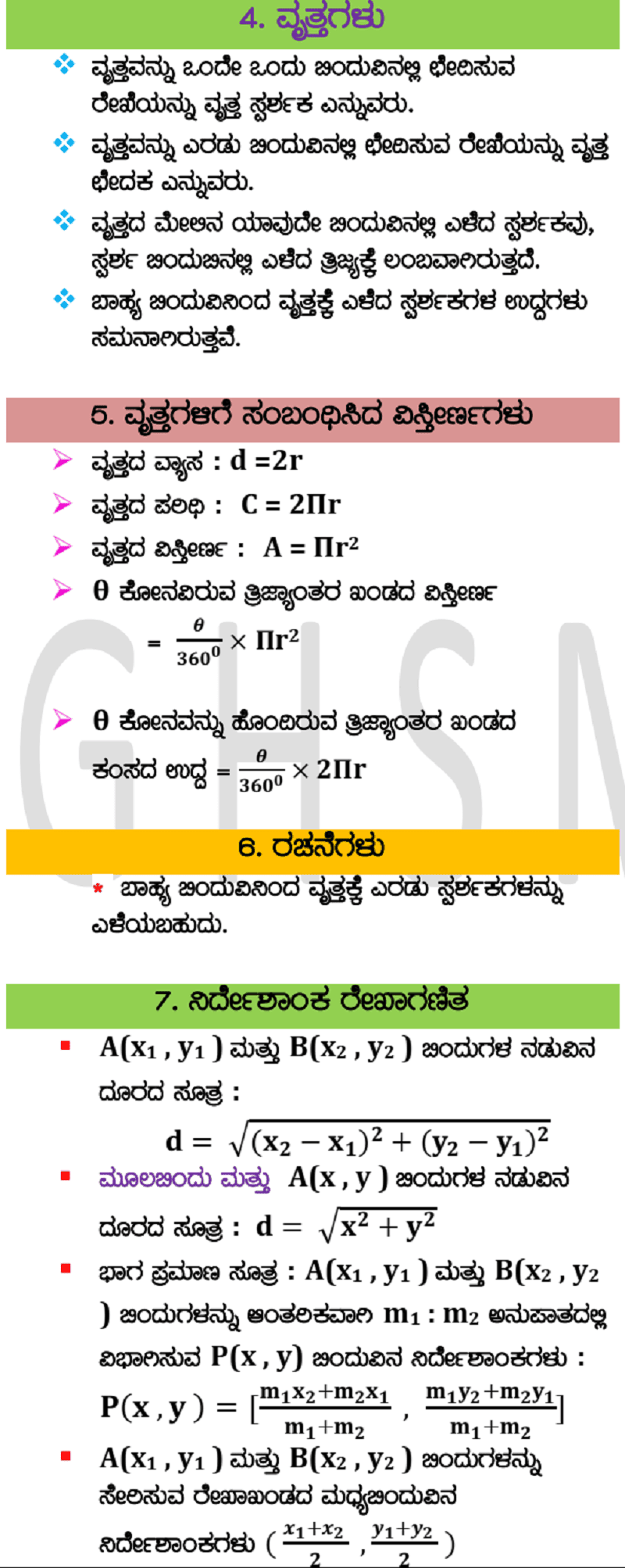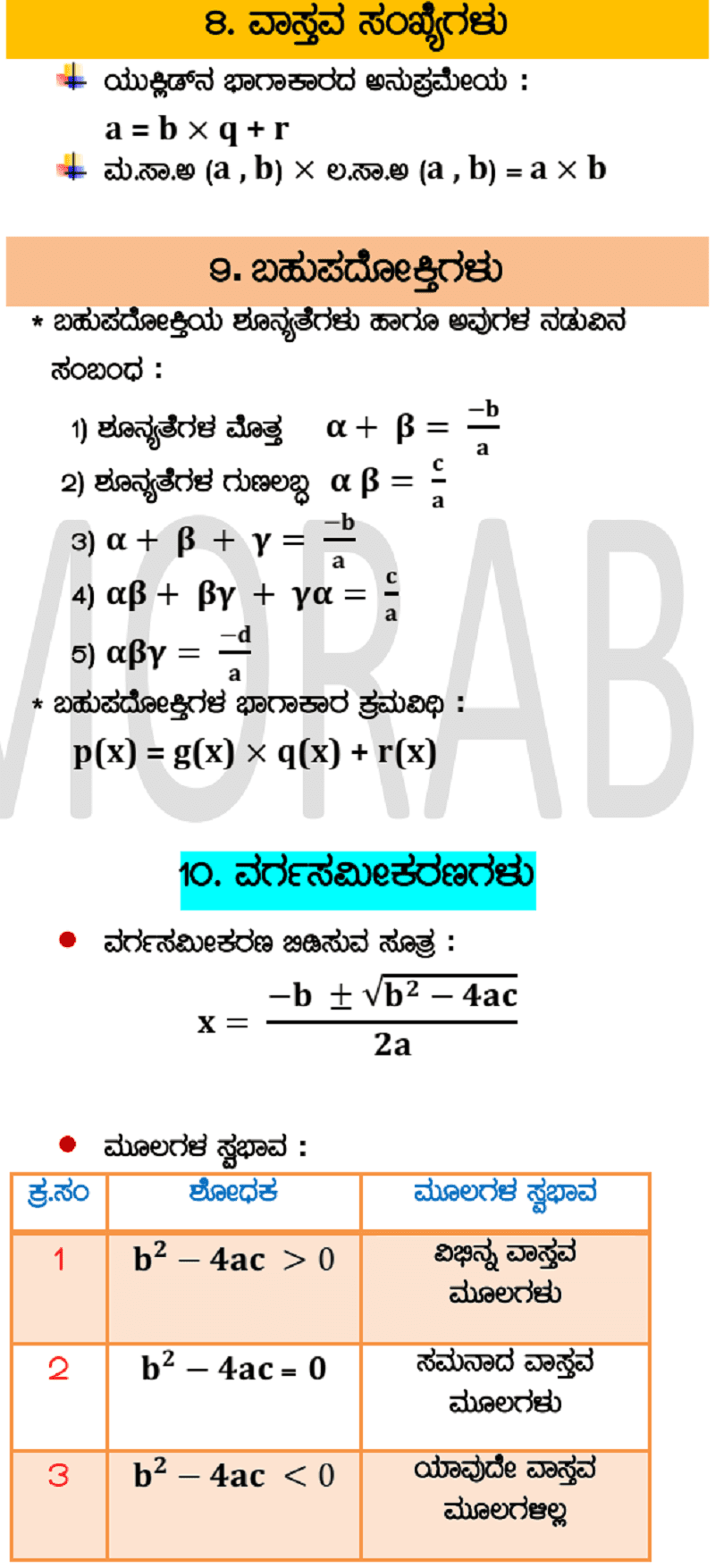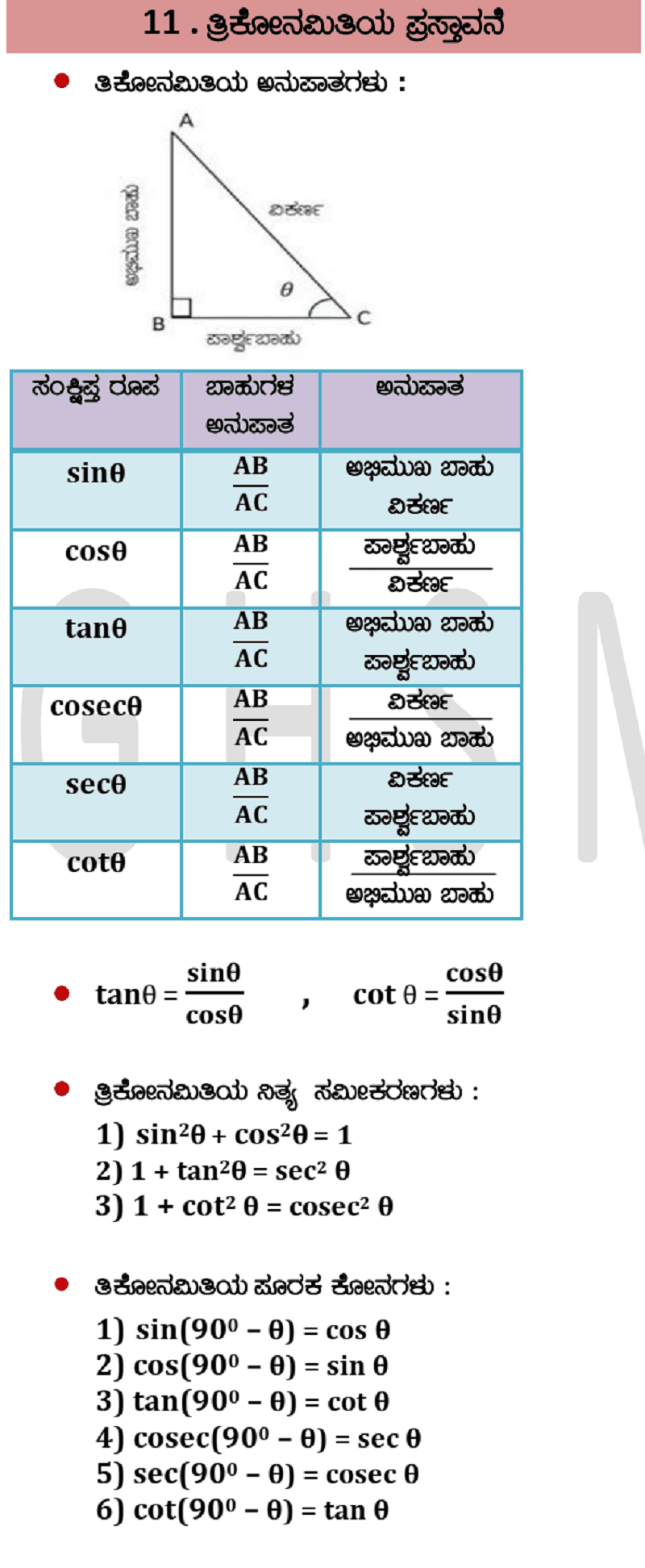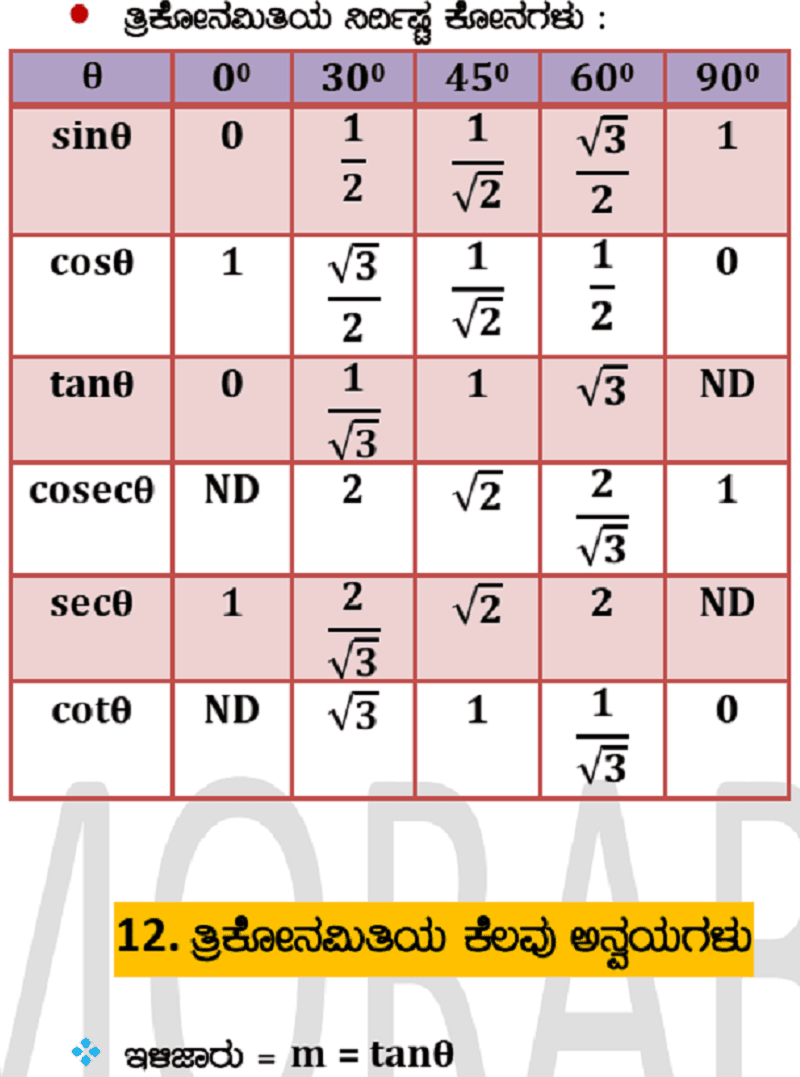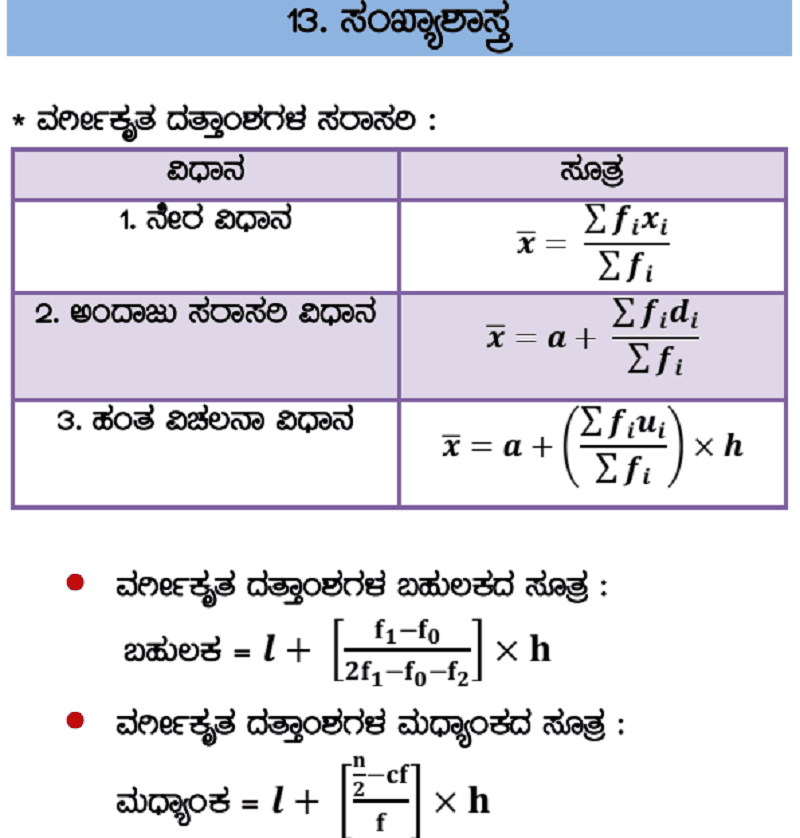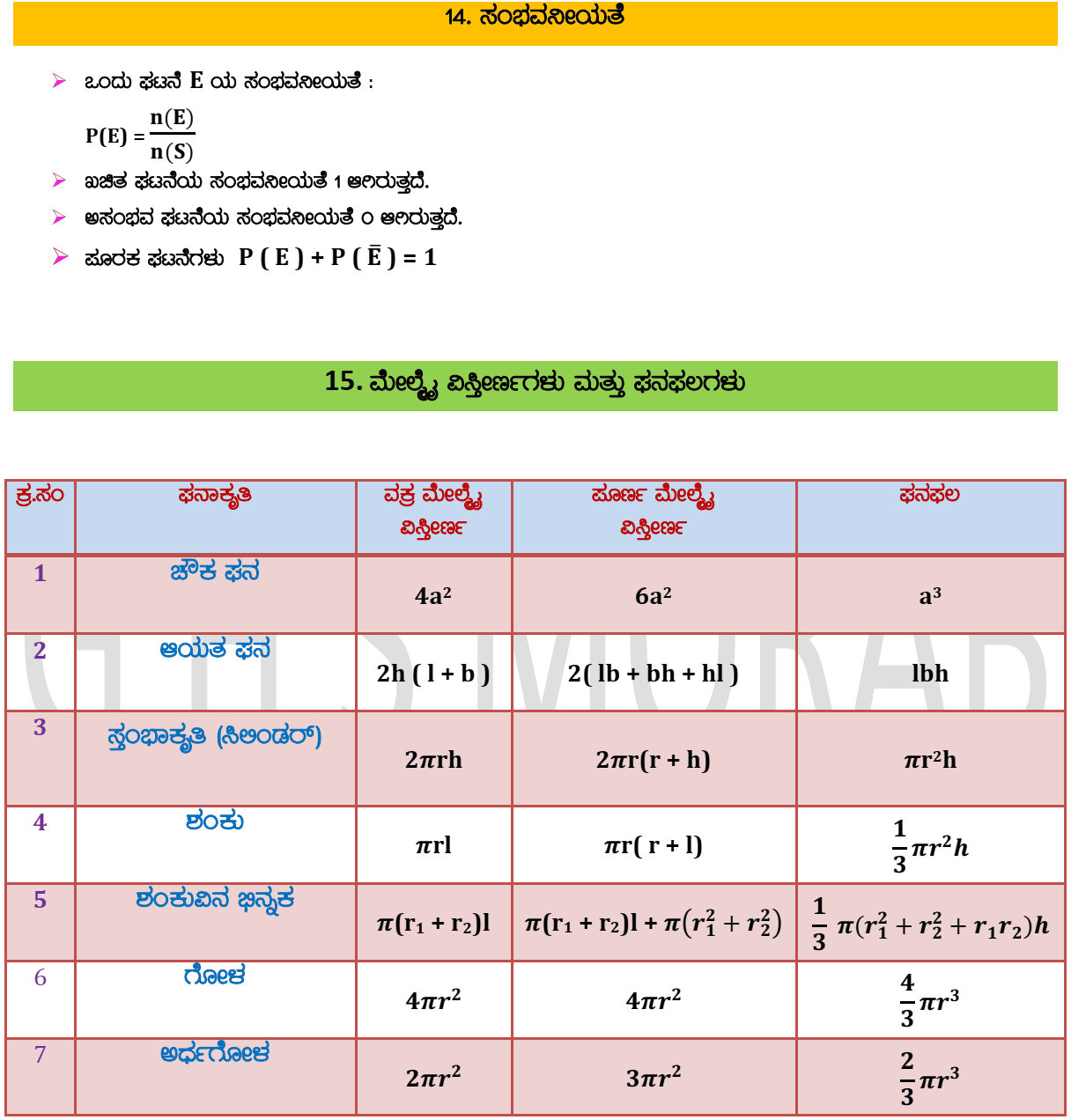# SSLC/10th Class Mathematics Formulas | All Chapters | Karnataka Board

SSLC/10th class is a crucial step in the academic journey of any student. Every student wants to score good numbers in the final exams. Many students feel that Mathematics is a kind of difficult subject and it requires lots of regular practice.

As you are very well aware that, every problem in Mathematics has the formula to solve it. Most of the time students are required to remember the Math formulas while solving Math problems. To help the students practice Math problems, we have a list of all the Mathematics formulas that can certainly be useful for the students who are preparing for the SSLC/10th examinations.

## SSLC/10th Class Mathematics Formulas: